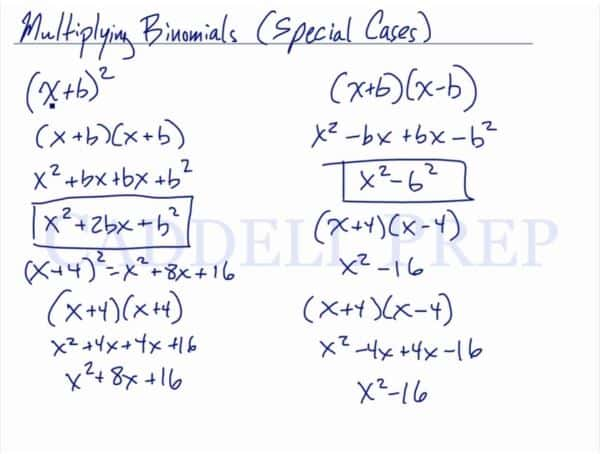In this video, we are going to look at how to multiply binomials with special cases. After you finish this lesson, view all of our Algebra 1 lessons and practice problems.

For example:
To multiply$(x+b)^2$ we have to multiply the binomial by itself. When we foil it, we get$x^2+bx+bx+b^2$
After combining like terms, this simplifies to$x^2+2bx+b^2$
By following this form, we can use this to solve any squared binomial.
For example:$(x+4)^2$
can be solved by plugging in 4 for where we see b. This will give us a final answer of$x^2+8x+16$
Another special case example would be to multiply$(x+b)(x-b)$
When we foil it we get$x^2-bx+bx-b^2$
After combining like terms, it simplifies to$x^2-b^2$
By following this form, we can use this to solve other binomials written this way.
For example:$(x+4)(x-4)$
would be easily solvable by plugging in 4 for where we see b. This will give us a final answer of$x^2-16$Example of Multiplying Binomials (Special Cases)

Example 1$Solve (x+9)^2$

We will follow the form$x^2+2bx+b^2$ to solve.$x^2+2(9)x+9^2$
Now, we have:$x^2+18x+81$

Example 2$Solve (x-11)(x+11)$

We will follow the form$x^2-b^2$ to solve.$x^2-11^2$
Now, we have:$x^2-121$

Video-Lesson Transcript

Let’s go over how to multiply binomials with special cases.

For example:$(x + b)^2$

This is multiplying a binomial by itself.

So what happens is this$(x + b) (x + b)$$x^2 + bx + bx + b^2$$x^2 + 2bx + b^2$

This means that you can immediately get the product of these two binomials if you follow this format.

For example:$(x + 4)^2$$(x + b)^2 = x^2 + 8x + 16$

I’ll do the math and check if that’s true.$(x + 4) (x + 4)$$x^2 + 4x + 4x + 16$

Combine like terms$x^2 + 8x + 16$

So, it’s supposed to come out like this.

Let’s have another special case.$(x + b) (x - b)$$x^2 - bx + bx - b^2$

So if we combine like terms, we’ll have$x^2 - b^2$

So if we have$(x + 4) (x - 4)$$x^2 - 16$

If we multiply it, we’ll have the same thing$(x + 4) (x - 4)$$x^2 - 4x + 4x - 16$$x^2 - 16$

Just like what we got earlier.

So in these special cases, just follow the format that we have above.